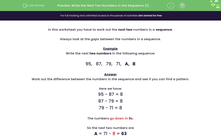# Write the Next Two Numbers in a Descending Sequence

In this worksheet, students will complete a descending number sequence by identifying the pattern.Key stage:  KS 2

Curriculum topic:   Number: Number and Place Value

Curriculum subtopic:   Solve Number Problems to 1000

Popular topics:   Ordering Numbers worksheets

Difficulty level:#### Worksheet Overview

In this activity, we will be working out the next two numbers in a sequence.

Always look at the gaps between the numbers in a sequence.

Example

Write the next two numbers in the following sequence:

95,   87,   79,   71,   A,   B

Work out the difference between the numbers in the sequence and see if you can find a pattern.

Here we have:

95 - 87 = 8

87 - 79 = 8

79 - 71 = 8

The numbers go down in 8s.

So the next two numbers are

A = 71 - 8 = 63

B = 63 - 8 = 55

95,   87,   79,   71,   63,   55

Be very careful with these descending sequences and remember we will need to subtract to find the missing numbers in the sequence.### What is EdPlace?

We're your National Curriculum aligned online education content provider helping each child succeed in English, maths and science from year 1 to GCSE. With an EdPlace account you’ll be able to track and measure progress, helping each child achieve their best. We build confidence and attainment by personalising each child’s learning at a level that suits them.

Get started